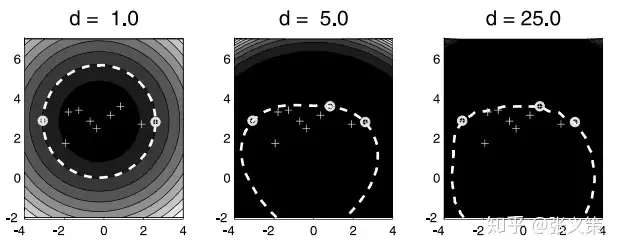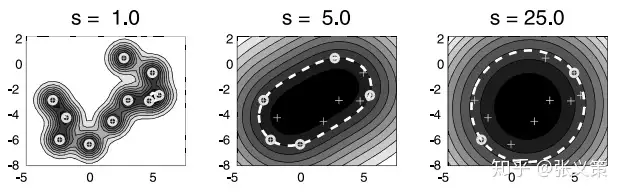### 简介

#### 单分类问题

• 单分类问题的目的并不时将不同类别的数据区分开来，而是对某个类别的数据生成一个描述（description），可以理解为是样本空间中的一个区域，当某个样本落在这个区域外，我们就认为该样本不属于这个类别。#### SVDD 思想

SVDD 的思路是寻找一个尽可能小的高维球体，将训练数据容纳进去，那么在预测数据时，认为在球体之外的数据为异常数据。

### 算法原理

#### 原始问题

• 假设我们有 $m$ 个样本点，分别为 $x(1),x(2),⋯,x(m)$ 。
• 我们假设这些样本点分布在一个球心为 $a$ ，半径为 $R$ 的球中。那么样本 $x(i)$ 满足
$$(x^{(i)}−a)^T(x^{(i)}−a)≤R^2$$
• 为了允许部分样本不在这个球中，我们引入松弛变量：
$$\left(x^{(i)}-a\right)^{T}\left(x^{(i)}-a\right) \leq R^{2}+\xi_{i}, \xi \geq 0$$
• 我们的目标是最小球的半径 $R$ 和松弛变量的值，于是目标函数:
$$\begin{array}{c} \min _{a, \xi,R} R^{2}+C \sum_{i=1}^{m} \xi_{i} \\ \text { s.t. }\left(x^{(i)}-a\right)^{T}\left(x^{(i)}-a\right) \leq R^{2}+\xi_{i}, \\ \quad \xi_{i} \geq 0, i=1,2, \cdots, m .\end{array}$$

• 写成拉格朗日方程：
\begin{aligned} L(R, a, \alpha, \xi, \gamma)=& R^{2}+C \sum_{i=1}^{m} \xi_{i} -\sum_{i=1}^{m} \alpha_{i}\left(R^{2}+\xi_{i}-\left(x^{(i)^{T}} x^{(i)}-2 a^{T} x^{(i)}+a^{2}\right)\right)-\sum_{i=1}^{m} \gamma_{i} \xi_{i} . \end{aligned}
• 其中， $α_i≥0,γ_i≥0$ 是拉格朗日乘子。

• 此时事实上涉及到了需要用 $\alpha$ 选择支持向量了，但是此时其他参数都还没有确定，那么确定那个样本作为支持向量更是无从谈起，因此原始问题：

$$\min_{R,a,\xi} \max_{\alpha,\gamma;\alpha\geq 0} L(R, a, \alpha, \xi, \gamma)$$

​ 无法求解，幸运的是该问题总体是凸优化问题，对偶问题与原问题等价，我们尝试求解对偶问题

#### 对偶问题

• 对偶问题：

$$\max_{\alpha,\gamma;\alpha\geq 0} \min_{R,a,\xi} L(R, a, \alpha, \xi, \gamma)$$

• 令拉格朗日函数对 $R,a,ξ_i$ 的偏导为 0，得到:
$$\begin{array}{c} \sum_{i=1}^{m} \alpha_{i}=1 \\ a=\sum_{i=1}^{m} \alpha_{i} x^{(i)} \\ C-\alpha_{i}-\gamma_{i}=0 \end{array}$$
• 我们可以将 $α_i$ 看作样本 $x^{(i)}$ 的权重。上式表明所有样本的权重之和为1，而球心 $a$ 是所有样本的加权和。将上式带入到拉格朗日函数中:
$$\begin{array}{c} \max _{\alpha} L(\alpha)=\sum_{i=1}^{m} \alpha_{i} x^{(i)^{T}} x^{(i)}-\sum_{i=1}^{m} \sum_{j=1}^{m} \alpha_{i} \alpha_{j} x^{(i)^{T}} x^{(j)}\\ s.t. 0 \leq \alpha_{i} \leq C \\ \sum_{i=1}^{m} \alpha_{i}=1, i=1,2, \cdots, m . \end{array}$$
• 当通过求解对偶问题得到 $α_i$ 后，可以通过 $a=\sum_{i=1}^{m} \alpha_{i} x^{(i)}$ 计算球心 $a$ 。
• 半径 $R$，则可以通过计算球心与支持向量（ $α_i<C$ ）之间的距离得到。当 $α_i=C$ 时，意味着样本$x^{(i)}$ 位于球的外面。

#### 判断新样本是否为异常点

• 对于一个新的样本点 $z$ ，如果它满足下式，那么我们认为它是一个异常点。
$$(z-a)^{T}(z-a)>R^{2}$$
• 展开上式，得:
$$z^{T} z-2 \sum_{i=1}^{m} \alpha_{i} z^{T} x^{(i)}+\sum_{i=1}^{m} \sum_{j=1}^{m} \alpha_{i} \alpha_{j} x^{(i)^{T}} x^{(j)}>R^{2}$$

### 引入核函数

• 只需将 ${K}\left(x^{(i)}, x^{(j)}\right)$ 替换 $x{(i){T}} x^{(j)}$ 即可。

• 在训练前通过变换函数$Φ:x→F$ 将数据从原始空间映射到新特征空间, 然后在新特征空间中导找一个体积最小的超球体, SVDD 要解决的优化问题变为:

$$\begin{array}{c} \min _{\mathbf{a}, R, \xi} R^{2}+C \sum_{i=1}^{n} \xi_{i}\\ s.t. \left\|\Phi\left(\mathbf{x}_{i}\right)-\mathbf{a}\right\|^{2} \leq R^{2}+\xi_{i}, \\\xi_{i} \geq 0, \forall i=1,2, \cdots, n \end{array}$$
• 那么对偶问题会变为
$$\begin{array}{c} \min _{\alpha_{i}} \sum_{i=1}^{n} \sum_{j=1}^{n} \alpha_{i} \alpha_{j} K\left(\mathbf{x}_{i}, \mathbf{x}_{j}\right)-\sum_{i=1}^{n} \alpha_{i} K\left(\mathbf{x}_{i}, \mathbf{x}_{i}\right) \\ s.t. \quad 0 \leq \alpha_{i} \leq C, \\ \sum_{i=1}^{n} \alpha_{i}=1 \\ \end{array}$$
• 求解该对偶问题后，可以获取所有样本对应的拉格朗日系数。
• 拉格朗日系数满足 $0<α_i<C$ 的样本称为支持向量，用 $\bf x_v$ 表示，那么球心和半径的公式为：
$$\mathbf{a}=\sum_{i=1}^{n} \alpha_{i} \Phi\left(\mathbf{x}_{i}\right)$$ $$R=\sqrt{K\left(\mathbf{x}_{v}, \mathbf{x}_{v}\right)-2 \sum_{i=1}^{n} \alpha_{i} K\left(\mathbf{x}_{v}, \mathbf{x}_{i}\right)+\sum_{i=1}^{n} \sum_{j=1}^{n} \alpha_{i} \alpha_{j} K\left(\mathbf{x}_{i}, \mathbf{x}_{j}\right)}$$

#### 多项式核

• 多项式核函数的表达式如下:
$$\mathcal {K} \left ( x ^ { (i) ^ {T} } x ^ {(j) } \right ) = (x ^ {(i) ^ {T}} x ^ {(j)}+1) ^ {d}$$
• 如下图所示，多项式核实际上不太适合 SVDD。特别是当 $d$ 取值非常大的时候。#### 高斯核

• 高斯核函数的表达式如下
$$\mathcal{K}\left(x^{(i)^{T}} x^{(j)}\right)=\exp (\frac{-\left(x^{(i)}-x^{(j)}\right)^{2}}{s^{2}})$$
• 如下图，相比于多项式核函数，高斯核函数的结果就合理多了。可以看到模型的复杂程度随着 $s$ 的增大而减小。### 参考资料

“觉得不错的话，给点打赏吧 ୧(๑•̀⌄•́๑)૭”https://www.zywvvd.com/notes/study/machine-learning/one-class/svdd/svdd/

Yiwei Zhang

2022年10月20日